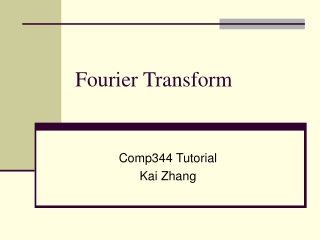DownloadDownload PresentationFourier Transform

# Fourier Transform

Download Presentation## Fourier Transform

- - - - - - - - - - - - - - - - - - - - - - - - - - - E N D - - - - - - - - - - - - - - - - - - - - - - - - - - -
##### Presentation Transcript

1. Fourier Transform Comp344 Tutorial Kai Zhang

2. Outline • Fourier Transform (FT) Properties • Fourier Transform of regular signals • Exercises

3. Popular forms of FT

4. Period and Frequency • Imagine a rod spinning around the center, taking 2 seconds for one round • Period • T = 2 (second) • Ordinary frequency • f = 0.5 (Hz or times/second) • Angular frequency • ω = 1 (degree/second) • Remember

5. FT Properties • Property 1: time domain shifting (or delay) • Proof

6. FT Properties • Property 2: frequency domain shifting • Proof

7. FT Properties • Property 3: scaling • Proof

8. FT Properties • Property 4: time domain differentiation • Proof • Question: what about

9. FT Properties • Property 5: Symmetry • Proof:

10. Some common FT-pairs • Impulse function

11. Some common FT-pairs • Complex exponential function • Using the frequency domain shifting property, and symmetry property

12. Some common FT-pairs • Sine function • By using the Euler formula and delay property

13. Some common FT-pairs • Rectangular function

14. Some common FT-pairs • Gaussian function

15. More Example • Let f(t)-F(w) be a FT-pair. Now compute the FT of g(t) = f(t)cos(t). • using Euler’s Formula and FT frequency shifting property

16. Exercise • Compute the FT of the following signals • u(t)cos(ω0t) • u(t)sin(ω0t)e-at • e-|a|t • u(t)e-at • u(t)te-at Here u(t) is the heavyside step function